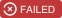# Group satellites into constellations such that their average observation coverage is maximizeddwave-examples, updated 🕥 2022-01-21 22:59:16# Satellite Placement

Suppose you have a set of `N` satellites and `k` targets on Earth that you want to observe. Each of your satellites has varying capabilities for Earth observation; in particular, the amount of ground that they can observe for a set amount of time is different. Since there are `k` targets, you would like to have `k` constellations to monitor said targets. How do you group your satellites into `k` constellations such that the coverage of each constellation is maximized? This is the question that we will be addressing in this demo!

There are two versions available. The first version has `N=12` and `k=4`. The larger version has `N=39` and `k=13`.

Note: in this demo we are assuming that `N` is a multiple of `k`.

## Usage

To run the smaller demo, using D-Wave's Simulated Annealing package (Neal), run the command:

```bash python satellite.py small.json neal```

To run the larger demo, using D-Wave's Hybrid Solver Service (HSS), run the command:

```bash python satellite.py large.json hss```

It will print out a set of satellite constellations and create an image to visualize the scores for each constellation, as shown below. Satellites closer to the center (grey star) have a higher score.Note: the larger demo is memory-intensive. It may use more than 10 GB of RAM.

## Code Overview

The idea is to consider all possible combinations of satellites, eliminate constellations with particularly low coverage, and encourage the following type of solutions:

• Constellations that have better coverage
• Satellites to only join one constellation
• A specific number of constellations in our final solution (i.e. encourage the solution to have `k` constellations)

## Code Specifics

We add weights to each constellation such that we are favoring constellations with a high coverage (aka high score). This is done with `bqm.add_variable(frozenset(constellation), -score)`. Note that `frozenset` is used to convey the concept that each variable in the model is a set of individual satellites, and the ordering does not matter. Incidentally, the code that defines the variables iterates over only the combinations (not the permutations), so defining the variables as tuples would work just as well (however, `set` cannot be used because the variables must be immutable).

## References

G. Bass, C. Tomlin, V. Kumar, P. Rihaczek, J. Dulny III. Heterogeneous Quantum Computing for Satellite Constellation Optimization: Solving the Weighted K-Clique Problem. 2018 Quantum Sci. Technol. 3 024010. https://arxiv.org/abs/1709.05381